# Chapter 3 Hedging Strategies using Futures Paul Koch

• Slides: 31Chapter 3: Hedging Strategies using Futures © Paul Koch 1 -1 A. Overview: Hedgers use futures to minimize risk. 1. What is the risk they are minimizing? The variance of the value of the unhedged position – how much uncertainty about the risk being taken. 2. Should you buy or sell futures to hedge your risk? Short Hedge: if you risk losing money when S↓ (example – Wheat Farmer – seller of wheat). Need to sell (short) futures. Long Hedge: if you risk losing money when S↑ (example – Wonder Bread – buyer of wheat). Need to buy (long) futures.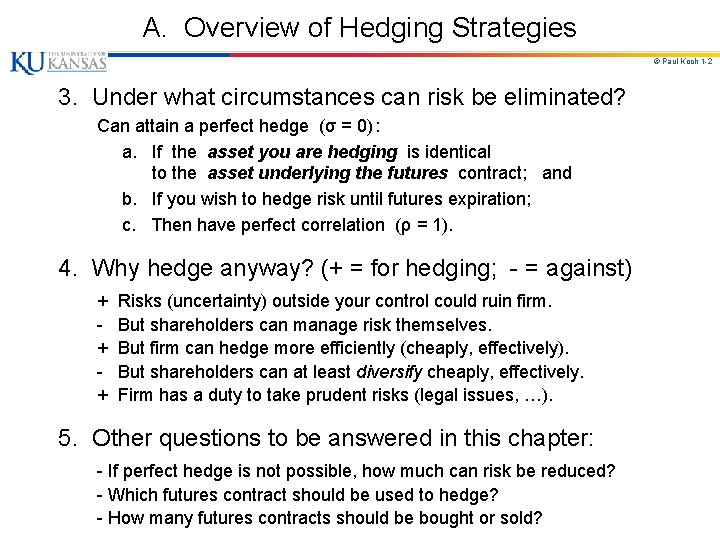A. Overview of Hedging Strategies © Paul Koch 1 -2 3. Under what circumstances can risk be eliminated? Can attain a perfect hedge (σ = 0) : a. If the asset you are hedging is identical to the asset underlying the futures contract; and b. If you wish to hedge risk until futures expiration; c. Then have perfect correlation (ρ = 1). 4. Why hedge anyway? (+ = for hedging; - = against) + Risks (uncertainty) outside your control could ruin firm. - But shareholders can manage risk themselves. + But firm can hedge more efficiently (cheaply, effectively). - But shareholders can at least diversify cheaply, effectively. + Firm has a duty to take prudent risks (legal issues, …). 5. Other questions to be answered in this chapter: - If perfect hedge is not possible, how much can risk be reduced? - Which futures contract should be used to hedge? - How many futures contracts should be bought or sold?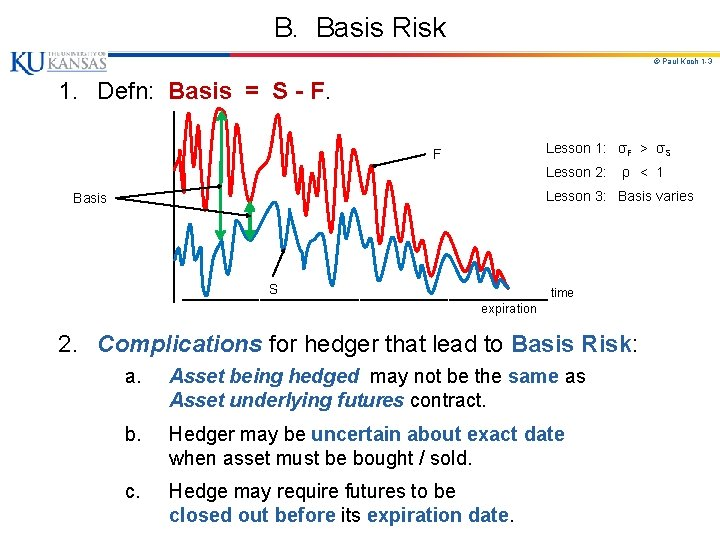B. Basis Risk © Paul Koch 1 -3 1. Defn: Basis = S - F. │ │ Lesson 1: σF > σS F │ Lesson 2: ρ < 1 │ Lesson 3: Basis varies │ │ │ S │_________________ time Basis expiration 2. Complications for hedger that lead to Basis Risk: a. Asset being hedged may not be the same as Asset underlying futures contract. b. Hedger may be uncertain about exact date when asset must be bought / sold. c. Hedge may require futures to be closed out before its expiration date.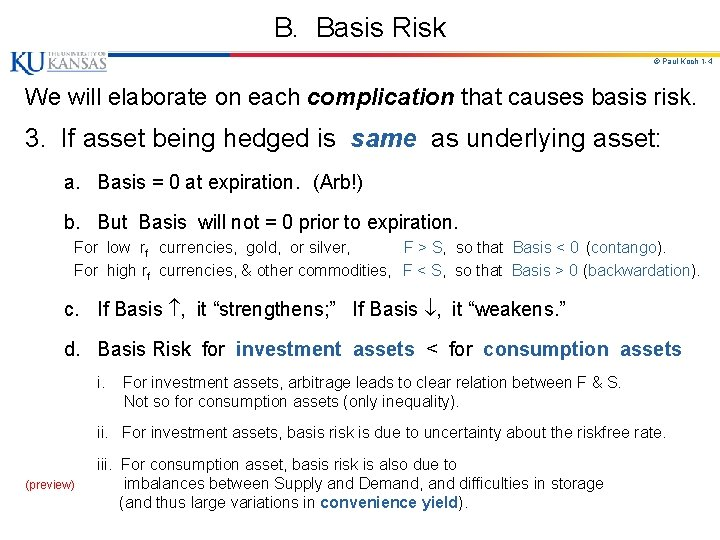B. Basis Risk © Paul Koch 1 -4 We will elaborate on each complication that causes basis risk. 3. If asset being hedged is same as underlying asset: a. Basis = 0 at expiration. (Arb!) b. But Basis will not = 0 prior to expiration. For low rf currencies, gold, or silver, F > S, so that Basis < 0 (contango). For high rf currencies, & other commodities, F < S, so that Basis > 0 (backwardation). c. If Basis , it “strengthens; ” If Basis , it “weakens. ” d. Basis Risk for investment assets < for consumption assets i. For investment assets, arbitrage leads to clear relation between F & S. Not so for consumption assets (only inequality). ii. For investment assets, basis risk is due to uncertainty about the riskfree rate. (preview) iii. For consumption asset, basis risk is also due to imbalances between Supply and Demand, and difficulties in storage (and thus large variations in convenience yield).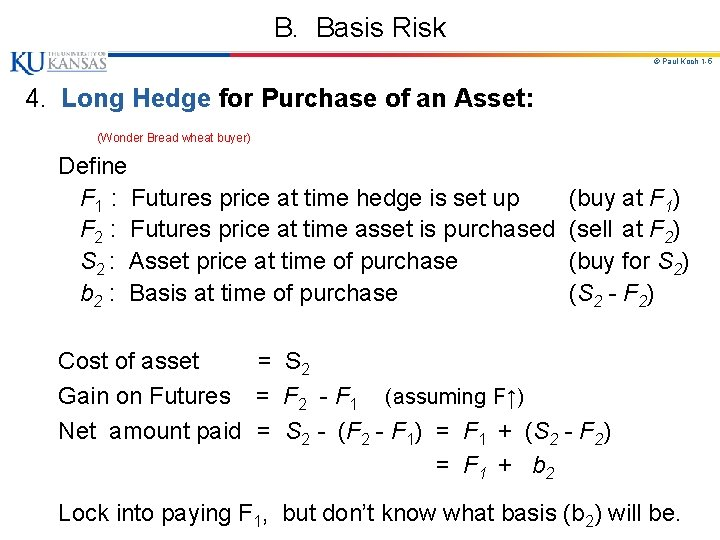B. Basis Risk © Paul Koch 1 -5 4. Long Hedge for Purchase of an Asset: (Wonder Bread wheat buyer) Define F 1 : Futures price at time hedge is set up (buy at F 1) F 2 : Futures price at time asset is purchased (sell at F 2) S 2 : Asset price at time of purchase (buy for S 2) b 2 : Basis at time of purchase (S 2 - F 2) Cost of asset = S 2 Gain on Futures = F 2 - F 1 (assuming F↑) Net amount paid = S 2 - (F 2 - F 1) = F 1 + (S 2 - F 2) = F 1 + b 2 Lock into paying F 1, but don’t know what basis (b 2) will be.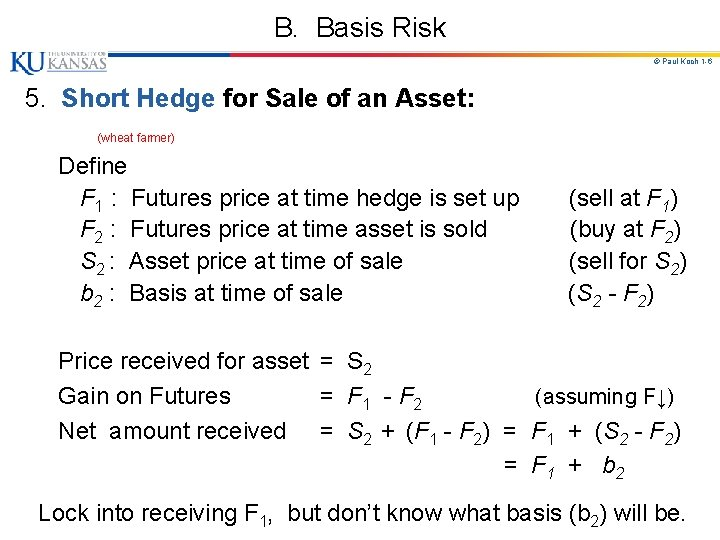B. Basis Risk © Paul Koch 1 -6 5. Short Hedge for Sale of an Asset: (wheat farmer) Define F 1 : Futures price at time hedge is set up (sell at F 1) F 2 : Futures price at time asset is sold (buy at F 2) S 2 : Asset price at time of sale (sell for S 2) b 2 : Basis at time of sale (S 2 - F 2) Price received for asset = S 2 Gain on Futures = F 1 - F 2 (assuming F↓) Net amount received = S 2 + (F 1 - F 2) = F 1 + (S 2 - F 2) = F 1 + b 2 Lock into receiving F 1, but don’t know what basis (b 2) will be.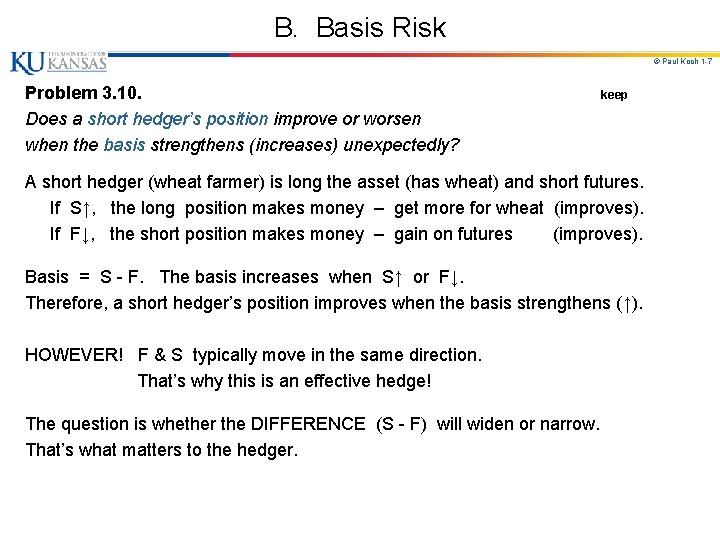B. Basis Risk © Paul Koch 1 -7 Problem 3. 10. Does a short hedger’s position improve or worsen when the basis strengthens (increases) unexpectedly? keep A short hedger (wheat farmer) is long the asset (has wheat) and short futures. If S↑, the long position makes money – get more for wheat (improves). If F↓, the short position makes money – gain on futures (improves). Basis = S - F. The basis increases when S↑ or F↓. Therefore, a short hedger’s position improves when the basis strengthens (↑). HOWEVER! F & S typically move in the same direction. That’s why this is an effective hedge! The question is whether the DIFFERENCE (S - F) will widen or narrow. That’s what matters to the hedger.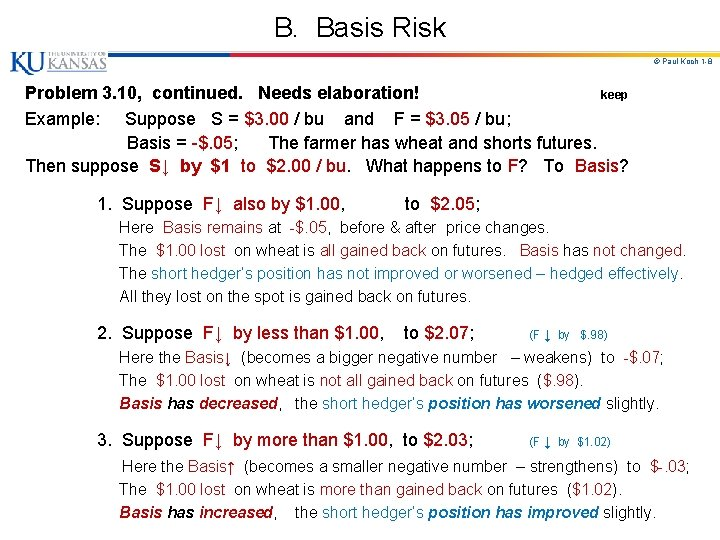B. Basis Risk © Paul Koch 1 -8 Problem 3. 10, continued. Needs elaboration! keep Example: Suppose S = \$3. 00 / bu and F = \$3. 05 / bu; Basis = -\$. 05; The farmer has wheat and shorts futures. Then suppose S↓ by \$1 to \$2. 00 / bu. What happens to F? To Basis? 1. Suppose F↓ also by \$1. 00, to \$2. 05; Here Basis remains at -\$. 05, before & after price changes. The \$1. 00 lost on wheat is all gained back on futures. Basis has not changed. The short hedger’s position has not improved or worsened – hedged effectively. All they lost on the spot is gained back on futures. 2. Suppose F↓ by less than \$1. 00, to \$2. 07; (F ↓ by \$. 98) Here the Basis↓ (becomes a bigger negative number – weakens) to -\$. 07; The \$1. 00 lost on wheat is not all gained back on futures (\$. 98). Basis has decreased, the short hedger’s position has worsened slightly. 3. Suppose F↓ by more than \$1. 00, to \$2. 03; (F ↓ by \$1. 02) Here the Basis↑ (becomes a smaller negative number – strengthens) to \$-. 03; The \$1. 00 lost on wheat is more than gained back on futures (\$1. 02). Basis has increased, the short hedger’s position has improved slightly.B. Basis Risk © Paul Koch 1 -9 6. If asset being hedged is not same as underlying asset. (For example, hedging spot price of jet fuel with heating oil futures. ) a. Cross-Hedge: Basis may not = 0 at expiration. b. Basis risk is usually greater. There is another component of basis risk. (No futures for jet fuel. ) Before, Basis = S - F. (e. g. , S for jet fuel - F for jet fuel) Now, Let S = spot price of asset being hedged (jet fuel); S* = spot price of asset underlying F (heating oil); Now there is clear relation between S* & F (both heating oil) rather than between S & F (jet fuel & oil). Add and subtract S*, and rearrange terms: Basis = (S* - F) + (S - S*); has another component.B. Basis Risk © Paul Koch 1 -10 7. For Cross-Hedge, choice of contract has 2 components: a. Choice of asset underlying the futures contract. i. If asset being hedged is same as that for futures, choice is easy. ii. Otherwise, pick F (and S*) most closely correlated with S. b. Choice of delivery month. i. May choose delivery month same as hedge expiration, or may choose later delivery month. ** ii. Usually choose next delivery month after hedge expiration: - F is often erratic during expiration month (more Basis risk). - long hedger runs risk of having to take delivery. - Basis risk increases as time between hedge expiration and delivery month increases. iii. This choice depends on liquidity of contracts with different maturities. - In practice, nearby contract is most liquid (low TC). - Hedger may choose short maturity futures, and roll them forward.C. Minimum Variance Hedge Ratio © Paul Koch 1 -11 Consider Cross-Hedge - if the assets are different. Deriving the Minimum Variance Hedge Ratio (h*). 1. Given that Basis may not = 0 at expiration, want to know its possible outcomes. a. What is mean and variance of Basis? Now “hedging” means managing the tails of this distribution. 2. Definition: Hedge Ratio (h). Let NA = # of units of asset held (the risk exposure); NF = # of units of (similar) asset hedged with futures. a. h = NF / NA = (size of futures position) (size of exposure). b. Up to now, have assumed h = 1 [amt hedged = amt exposed].C. Minimum Variance Hedge Ratio © Paul Koch 1 -12 3. As we vary h, the variance of hedger's position changes. a. h* = value of h that minimizes the variance of hedger's position. b. If objective is to minimize risk, h* may not = 1!C. Minimum Variance Hedge Ratio © Paul Koch 1 -13 4. The Framework. Consider a short hedge ( long St , short h x Ft ). Focus on combined hedged position in asset and futures. Derive minimum variance hedge ratio (h*). a. Notation: St = Spot price of asset at time t (t = 1, 2). Ft = Futures price at time t. ΔS = Change in S during period of hedge (S 2 - S 1). ΔF = Change in F " " " " (F 2 - F 1). σS = Standard deviation of ΔS. σF = Standard deviation of ΔF. ρ = Correlation between ΔS and ΔF. b. Definitions: Ct = St - h Ft = combined hedged position; [Long the asset (St) and short h futures (Ft), where h = hedge ratio. ] Note: Ct = St - h Ft , and: h* = ρ(σS / σF) = optimal hedge ratio min Var( Ct).C. Minimum Variance Hedge Ratio © Paul Koch 1 -14 c. Derivation of formula for h*: Want to minimize uncertainty about combined hedged position; Want to minimize VC = Var( Ct) = Var [ ΔS - h ΔF ]. // \ Digression: Var [ a. X + b. Y ] = a 2 σX 2 + b 2 σY 2 + 2 ab(ρ σX σY). \ // Var [ ΔS - h ΔF ] = σS 2 + h 2σF 2 - 2 h(ρ σS σF) ---(parabola in h) Var [ ΔS - h ΔF ] / h = 2(h*)σF 2 - 2(ρ σS σF) = 0 Solving for h*: h* = (ρ σS σF) / σF 2 ╔══════╗ or: ║ h* = ρ(σS / σF) ║ ╚══════╝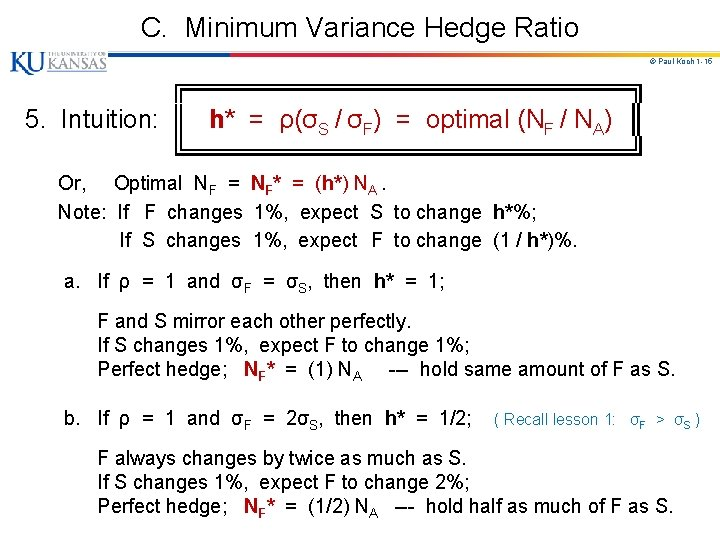C. Minimum Variance Hedge Ratio © Paul Koch 1 -15 ╔════════════╗ 5. Intuition: ║ h* = ρ(σS / σF) = optimal (NF / NA) ║ ╚════════════╝ Or, Optimal NF = NF* = (h*) NA. Note: If F changes 1%, expect S to change h*%; If S changes 1%, expect F to change (1 / h*)%. a. If ρ = 1 and σF = σS, then h* = 1; F and S mirror each other perfectly. If S changes 1%, expect F to change 1%; Perfect hedge; NF* = (1) NA --- hold same amount of F as S. b. If ρ = 1 and σF = 2σS, then h* = 1/2; ( Recall lesson 1: σF > σS ) F always changes by twice as much as S. If S changes 1%, expect F to change 2%; Perfect hedge; NF* = (1/2) NA --- hold half as much of F as S.C. Minimum Variance Hedge Ratio © Paul Koch 1 -16 c. ╔══════╗ ║ h* = ρ(σS / σF) ║ ╚══════╝ If ρ < 1, then h* depends on magnitude of ρ and ratio, σS / σF. Intuition still holds true. If S changes 1%, expect F to change (1 / h*)%; Imperfect hedge; NF* = h*NA --- hold h* as much F as S. d. Example: If h* =. 786, futures position should have 78. 6% of face value of asset being hedged. If S changes 1%, expect F to change more (1 /. 786)%; Imperfect hedge; if you hold NF* = (. 786) NA, the value of your futures position is expected to just offset the change in S.C. Minimum Variance Hedge Ratio © Kelly D. Welch 1 -17 e. ╔══════╗ ║ h* = ρ(σS / σF) ║ ╚══════╝ To minimize risk, should the hedger hold more or less futures, compared to the amount held of the underlying risk exposure? Is h* > 1 or < 1? Lesson 1: σF > σS Lesson 2: ρ < 1 This means: h* = ρ(σS / σF) < 1 for most situations.C. Minimum Variance Hedge Ratio © Paul Koch 1 -18 f. h* is slope of best fit line when ΔS is regressed on ΔF. In practice, get data on ΔS & ΔF, measured over hedge horizon. Regression coefficient is hedge ratio, h*. ΔS │ ● ● │ ● ● │● ● ● │ ● ● ● │ ● ● ● │ ● ● ──────────────────────── ΔF ● ● ● │ ● ● ● │ ● ● ● │● Lesson 1: σF > σS ● │ Lesson 2: ρ < 1 ● ● ● │ ● ● │ Slope = h* = ρ(σS / σF) < 1 ● │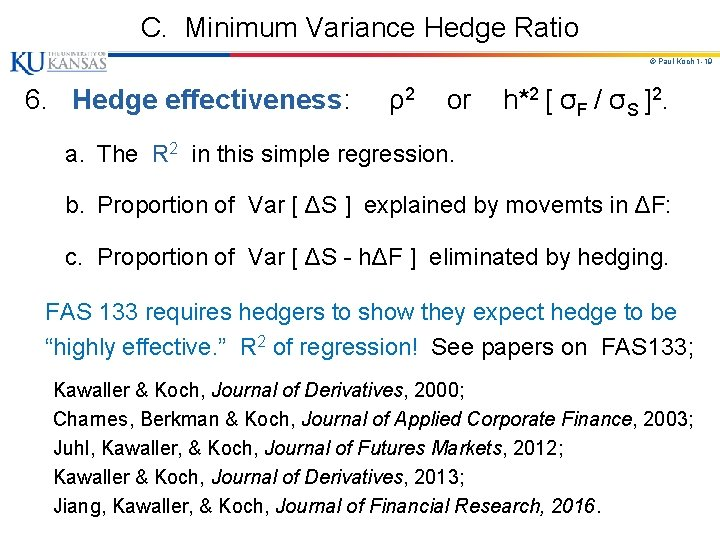C. Minimum Variance Hedge Ratio © Paul Koch 1 -19 6. Hedge effectiveness: ρ2 or h*2 [ σF / σS ]2. a. The R 2 in this simple regression. b. Proportion of Var [ ΔS ] explained by movemts in ΔF: c. Proportion of Var [ ΔS - hΔF ] eliminated by hedging. FAS 133 requires hedgers to show they expect hedge to be “highly effective. ” R 2 of regression! See papers on FAS 133; Kawaller & Koch, Journal of Derivatives, 2000; Charnes, Berkman & Koch, Journal of Applied Corporate Finance, 2003; Juhl, Kawaller, & Koch, Journal of Futures Markets, 2012; Kawaller & Koch, Journal of Derivatives, 2013; Jiang, Kawaller, & Koch, Journal of Financial Research, 2016.C. Minimum Variance Hedge Ratio © Paul Koch 1 -20 7. Optimal number of futures contracts (N*). a. Define: NA = size of position being hedged (units); QF = size of one futures contract (units). Recall, h* = NF* / NA; or, NF* = (h*) NA. The futures hedge should have a face value of (h*) NA. Thus, get N* by simply dividing this face value (NF*) by QF: ╔══════════════╗ ║ N* = [ (h*) NA ] / QF = [ (ρσS / σF) NA ] / QF ║ ╚══════════════╝ b. In practice, # of futures contracts must be an integer; Thus, hedger can only approximate optimal hedge.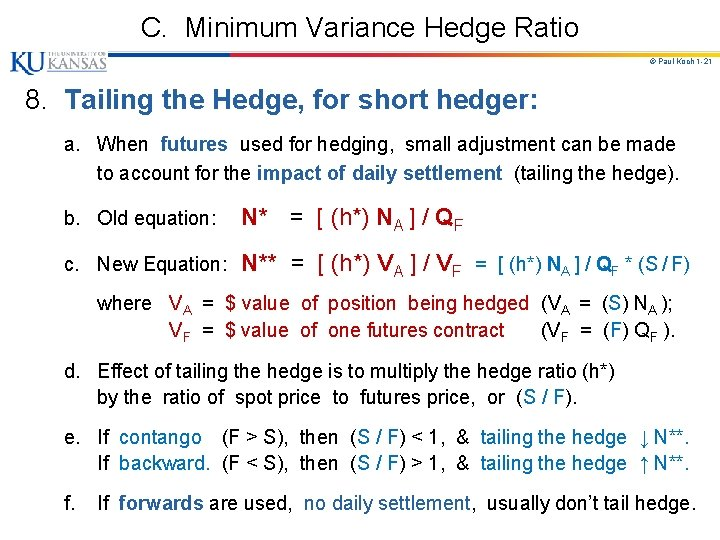C. Minimum Variance Hedge Ratio © Paul Koch 1 -21 8. Tailing the Hedge, for short hedger: a. When futures used for hedging, small adjustment can be made to account for the impact of daily settlement (tailing the hedge). b. Old equation: N* = [ (h*) NA ] / QF c. New Equation: N** = [ (h*) VA ] / VF = [ (h*) NA ] / QF * (S / F) where VA = \$ value of position being hedged (VA = (S) NA ); VF = \$ value of one futures contract (VF = (F) QF ). d. Effect of tailing the hedge is to multiply the hedge ratio (h*) by the ratio of spot price to futures price, or (S / F). e. If contango (F > S), then (S / F) < 1, & tailing the hedge ↓ N**. If backward. (F < S), then (S / F) > 1, & tailing the hedge ↑ N**. f. If forwards are used, no daily settlement, usually don’t tail hedge.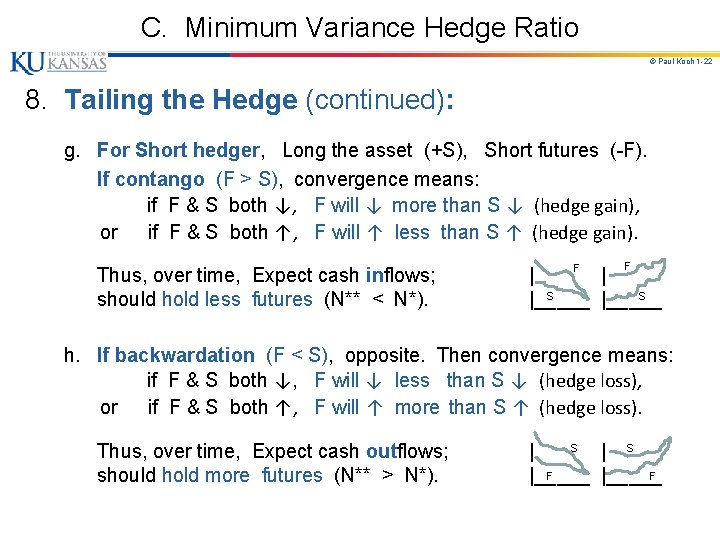C. Minimum Variance Hedge Ratio © Paul Koch 1 -22 8. Tailing the Hedge (continued): g. For Short hedger, Long the asset (+S), Short futures (-F). If contango (F > S), convergence means: if F & S both ↓, F will ↓ more than S ↓ (hedge gain), or if F & S both ↑, F will ↑ less than S ↑ (hedge gain). Thus, over time, Expect cash inflows; should hold less futures (N** < N*). F F | | S S |_____ h. If backwardation (F < S), opposite. Then convergence means: if F & S both ↓, F will ↓ less than S ↓ (hedge loss), or if F & S both ↑, F will ↑ more than S ↑ (hedge loss). Thus, over time, Expect cash outflows; should hold more futures (N** > N*). S | | S F F |_____C. Minimum Variance Hedge Ratio © Paul Koch 1 -23 9. Example: Cross-Hedge; (no daily settlemt; no tailing) § Airline uses Heating Oil forwards to hedge Jet Fuel costs. § Airline will purchase 2 million gallons of Jet Fuel in 1 month, and hedges by buying xxxx gallons of Heating Oil forward. § From historical data s. F = 0. 0313, s. S = 0. 0263, & r = 0. 928 Airline should buy forward (. 7777) x (2, 000 gal) = 1, 555, 400 gal (fwd 1 mo). § Or, if they use Heating Oil futures: 1 contract = 42, 000 gal. N* = [ (h*) NA ] / QF = [ (. 7777) x (2, 000 gal )] / (42, 000 gal) = 37. 03 contracts. The Airline would buy 37 futures contracts (if no tailing).C. Minimum Variance Hedge Ratio © Paul Koch 1 -24 10. Example: Cross-Hedge; (daily settlemt; tailing hedge) § If Airline uses Heating Oil futures to hedge Jet Fuel costs. § From historical data s. F = 0. 0313, s. S = 0. 0263, & r = 0. 928 § The size of one Heating Oil contract is 42, 000 gal. § Spot price = S = \$1. 94 / gal; Futures price = F = \$1. 99 / gal. § Optimal number of contracts after tailing the hedge: N** = [ (h*) VA ] / VF = [ (h*) NA ] / QF x (S / F) = [ (. 7777) x (\$1. 94 x 2, 000 gal ) ] / (\$1. 99 x 42, 000 gal ) = 36. 10 contracts. (Note: S < F, (S / F) < 1; N** ↓) The Airline should buy 36 contracts.D. Hedging a Stock Portfolio © Paul Koch 1 -25 Hedging stock portfolio with Stock Index Futures. 1. Define: VA = Current value of stock portfolio; VF = Current value of one futures contract. 2. If stock portfolio perfectly matches the index (β = 1), Optimal Number of Futures Contracts = N* = VA / VF. a. Example: VA = \$1, 000; Index Value = 1, 000; VF = \$250 x Index = \$250, 000; Short N* = VA / VF = \$1, 000 / \$250, 000 = 4 contracts. ( Value of 4 contracts matches Value of stock portfolio. )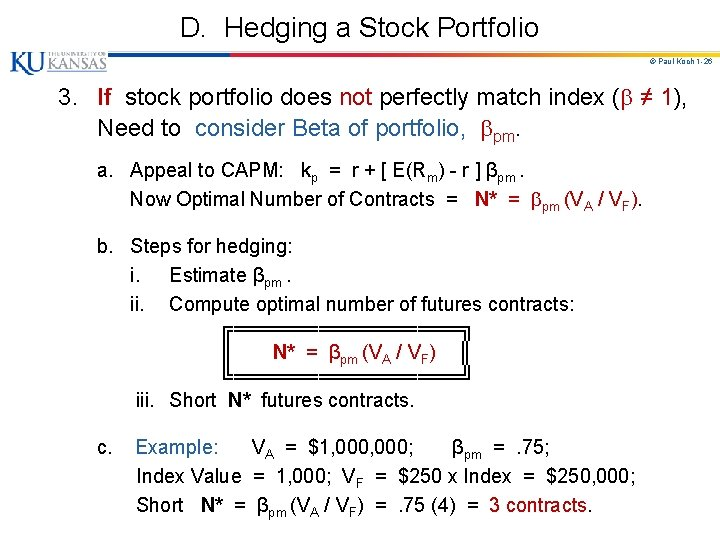D. Hedging a Stock Portfolio © Paul Koch 1 -26 3. If stock portfolio does not perfectly match index ( ≠ 1), Need to consider Beta of portfolio, pm. a. Appeal to CAPM: kp = r + [ E(Rm) - r ] βpm. Now Optimal Number of Contracts = N* = pm (VA / VF). b. Steps for hedging: i. Estimate βpm. ii. Compute optimal number of futures contracts: ╔════════╗ ║ N* = βpm (VA / VF) ║ ╚════════╝ iii. Short N* futures contracts. c. Example: VA = \$1, 000; βpm = . 75; Index Value = 1, 000; VF = \$250 x Index = \$250, 000; Short N* = βpm (VA / VF) = . 75 (4) = 3 contracts.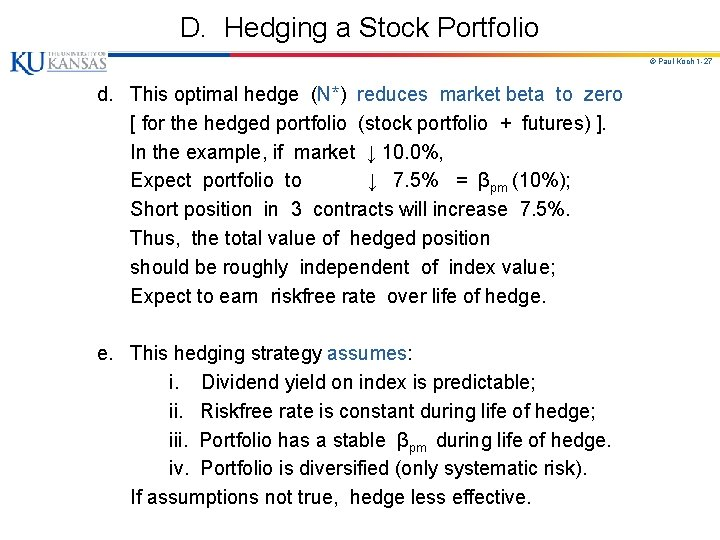D. Hedging a Stock Portfolio © Paul Koch 1 -27 d. This optimal hedge (N*) reduces market beta to zero [ for the hedged portfolio (stock portfolio + futures) ]. In the example, if market ↓ 10. 0%, Expect portfolio to ↓ 7. 5% = βpm (10%); Short position in 3 contracts will increase 7. 5%. Thus, the total value of hedged position should be roughly independent of index value; Expect to earn riskfree rate over life of hedge. e. This hedging strategy assumes: i. Dividend yield on index is predictable; ii. Riskfree rate is constant during life of hedge; iii. Portfolio has a stable βpm during life of hedge. iv. Portfolio is diversified (only systematic risk). If assumptions not true, hedge less effective.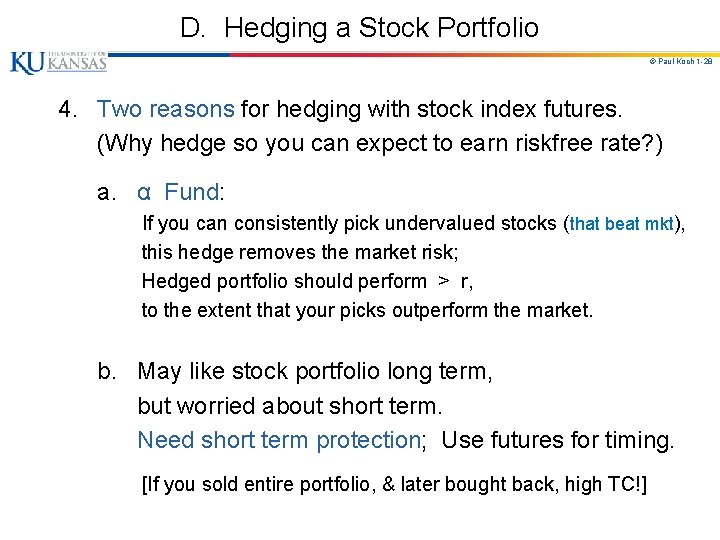D. Hedging a Stock Portfolio © Paul Koch 1 -28 4. Two reasons for hedging with stock index futures. (Why hedge so you can expect to earn riskfree rate? ) a. α Fund: If you can consistently pick undervalued stocks (that beat mkt), this hedge removes the market risk; Hedged portfolio should perform > r, to the extent that your picks outperform the market. b. May like stock portfolio long term, but worried about short term. Need short term protection; Use futures for timing. [If you sold entire portfolio, & later bought back, high TC!]D. Hedging a Stock Portfolio © Paul Koch 1 -29 5. Can change beta of portfolio to anything desired. a. N* = βpm (VA / VF) contracts reduce β from βpm to zero. b. N* / 2 contracts reduce β from βpm to βpm / 2. c. In general, can change β from βpm to any β* desired: i. If βpm > β*, short (βpm - β*) (VA / VF) contracts; ii. If βpm < β*, long (β* - βpm) (VA / VF) contracts. 6. Can use stock index futures to hedge individual stock. a. Special case of 5. above, where βpm = βim. N* = βpm (VA / VF) contracts reduce β of stock from βim to 0. b. Note: N* is calculated same way, but performance worse! This hedge only protects against systematic (market) risk, not unsystematic risk in individual stock. (See Prob. 3. 18)E. Stack and Roll © Paul Koch 1 -30 1. Expiration date of the hedge may be later than delivery dates of all usable (liquid) futures contracts. User chooses short term (nearby) futures contract to hedge until it expires; Then rolls hedge forward; as futures expire, close one & open another. Procedure: company expects to receive price in future at time Tn; T 0: Short futures contract #1 expiring at T 1; T 1: Close out futures contract #1; Short futures contract #2 expiring at T 2; T 2: Close out futures contract #2; Short futures contract #3 expiring at T 3; . . . Tn-1: Tn: Close out futures contract #n-1; Short futures contract #n expiring at Tn; Close out futures contract #n. Will not be perfect hedge. Outcome depends on futures prices (& Basis!) you’ll get at times T 1, T 2, …, Tn. If market moves against, margin calls!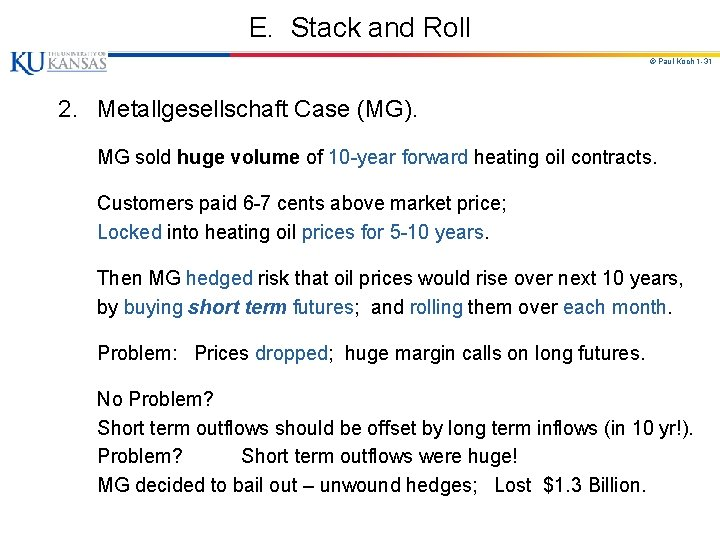E. Stack and Roll © Paul Koch 1 -31 2. Metallgesellschaft Case (MG). MG sold huge volume of 10 -year forward heating oil contracts. Customers paid 6 -7 cents above market price; Locked into heating oil prices for 5 -10 years. Then MG hedged risk that oil prices would rise over next 10 years, by buying short term futures; and rolling them over each month. Problem: Prices dropped; huge margin calls on long futures. No Problem? Short term outflows should be offset by long term inflows (in 10 yr!). Problem? Short term outflows were huge! MG decided to bail out – unwound hedges; Lost \$1. 3 Billion.